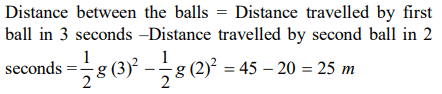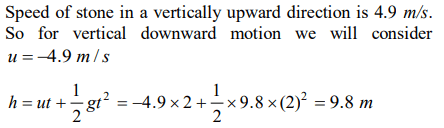## Motion in a Straight Line Questions and Answers Part-16

1. A body starts to fall freely under gravity. The distances covered by it in first, second and third second are in ratio
a) $1:3:5$
b) $1:2:3$
c) $1:4:9$
d) $1:5:6$

Explanation:2. P, Q and R are three balloons ascending with velocities U, 4U and 8U respectively. If stones of the same mass be dropped from each, when they are at the same height, then
a) They reach the ground at the same time
b) Stone from P reaches the ground first
c) Stone from R reaches the ground first
d) Stone from Q reaches the ground first

Explanation: It has lesser initial upward velocity.

3. A body is projected up with a speed 'u' and thetime taken by it is T to reach the maximum height H . Pick out the correct statement
a) It reaches H / 2 in T / 2 sec
b) It acquires velocity u / 2 in T / 2 sec
c) Its velocity is u / 2 at H / 2
d) Same velocity at 2T

Explanation:4. A body falling for 2 seconds covers a distance S equal to that covered in next second. Taking $g=10 m \diagup s^{2},S=$
a) 30 m
b) 10 m
c) 60 m
d) 20 m

Explanation: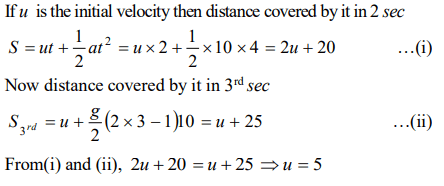5. A body dropped from a height h with an initial speed zero, strikes the ground with a velocity $3 km \diagup h$ . Another body of same mass is dropped from the same height h with an initial speed $-u' = 4km\diagup h$  . Find the final velocity of second body with which it strikes the ground
a) $3km\diagup h$
b) $4km\diagup h$
c) $5km\diagup h$
d) $12km\diagup h$

Explanation:6. A ball of mass $m_{1}$ and another ball of mass m2 are dropped from equal height. If time taken by the balls are $t_{1}$ and $t_{2}$ respectively, then
a) $t_{1}=\frac{t_{2}}{2}$
b) $t_{1}=t_{2}$
c) $t_{1}=4t_{2}$
d) $t_{1}=\frac{t_{2}}{4}$

Explanation: The time of fall is independent of the mass

7. With what velocity a ball be projected vertically so that the distance covered by it in 5th second is twice the distance it covers in its 6th second ($g=10 m \diagup s^{2}$  )
a) 58.8 m/s
b) 49 m/s
c) 65 m/s
d) 19.6 m/s

Explanation: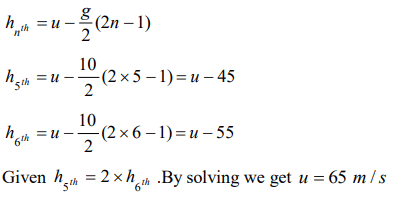8. A body sliding on a smooth inclined plane requires 4 seconds to reach the bottom starting from rest at the top. How much time does it take to cover one-fourth distance starting from rest at the top
a) 1 s
b) 2 s
c) 4 s
d) 16 s

Explanation: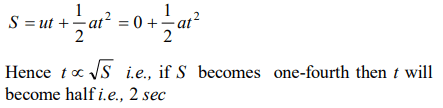9. A ball is dropped downwards. After 1 second another ball is dropped downwards from the same point. What is the distance between them after 3 seconds
a) 25 m
b) 20 m
c) 50 m
d) 9.8 m